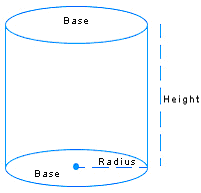# Cylinders, Cones and Spheres

### The Basics

We are familiar with the shapes of a number of polyhedrons like cubes, cuboids and pyramids but now we will study about cylinders, cones and spheres, which are not polyhedrons. Cylinders, cones, and spheres are not polyhedrons, since they have curved and not flat surfaces.

In our daily life, we come across a number of solids, which are either a part of such a solid or a combination of them. For example, a circular pillar has the shape of a cylinder. A glass tumbler is a part of a right circular cone and a conical circus tent with a cylindrical base is a combination of a right circular cylinder and a right circular cone

A cylinder is similar to prism but has a circular cross section. In right cylinder, the curved surface is perpendicular to the base. A cone is a pyramid with a circular cross section. A right cone is a cone with its vertex above the center of its base. The sphere is a space figure, which all its points at an equal distance from the center point.

### Details:

In this unit, we discuss those space figures, which are not polyhedrons.Â  Let us begin with figures like Cylinders, Cones, and Spheres. These figures have curved, not flat, surfaces unlike polyhedrons.

In this lesson, we study some common space figures that are not polyhedrons. These figures have some things in common like they all have some curved surfaces, while the surfaces of a polyhedron are always flat.

ÂÂ

Cylinder: The cylinder is somewhat like a prism with parallel congruent circular bases. Notice an ordinary circular lead pencil or a water pipe. A right circular cylinder is a solid generated by the revolution of a rectangle about one of its sides. You can find the volume of a cylinder the same way as you do in the case of a prism i.e. base area multiplied by the height of a cylinder.
V = B x H

Where V stands for volume, B for base and H for height.

As, we know that the base of a cylinder is circle, we can put the area of a circle (r2) replacing the base (B) in the formula of a volume:

Thus, the formula for the Volume of a Cylinder is:

V =r2 x H
=r2h

Where, r is the radius of the base and h is the height of the cylinder

ÂCone:Â  Right circular cone is a solid generated by revolving a right triangle about one of the sides containing the right angle.Â  A cone is similar in some ways to a pyramid since it has one circular base and converges to meet at a point called vertex. The formula for the volume of a cone is

V= 1/3 (B x H)

Â Where V stands for volume, B stands for the base and H stands for height.

As the base of a cone is of circular shape, we can put the area of a circle (r2) instead of base (B) in the formula of a volume:

V = 1/3 (r2 x H)Sphere:Â  A sphere is a set of points in a space whose distance from a fixed point is equal to a given distance. The fixed point is called the centre and the given distance is called the radius of the sphere. Glass marbles used for play, small metal balls used in cycle bearings, soccer balls, iron shot used for shot-put are all examples of spheres. A sphere has a radius and a diameter like circle. Even the shape of the earth is like a large sphere. The formula for volume of sphere is

V = 4/3 (r3)

### Examples

Problem:

Find the volume of a cylinder shaped can with a height of 9 inches and a diameter of 8 inches:

Solution:

When the diameter is 8 inches, the radius would be 4 inches. Thus, applying the volume formula of a cylinder, we would get:

V =r2h
or, V = 3.14 (42 x 9)
or, V = 3.14 (16 x 9)
or, V = 3.14 x 144
or, V = 452.16 inch3

Thus, Volume of the cylinder = 452.16 inch3

Problem:

Find the volume of a cone with a height of 6 inches and a radius of 4 inches:

Solution:

Thus, applying the volume formula of a cone, we would get:

V = 1/3 (r2 x H)
or, V = 1/3 (3.14 x 42 x 6)
or, V =Â  1/3 (3.14 x 96)
or, V = 1/3 (301.44)
or, V = 100.48 inch3

Thus, Volume of the cone = 100.48 inch3

Problem:

Find the volume of a sphere shaped soccer ball with a diameter of 8 inches:

Solution:

When the diameter is 8 inches, the radius would be 4 inches. Thus, applying the volume formula of a sphere, we would get:

V = 4/3 (r3)
or, V = 4/3 (3.14 x 43)
or, V = 4/3 (3.14 x 64)
or, V = 4/3 xÂ  200.96
or, V = 267.94 inch3

Thus, Volume of the sphere = 267.94 inch3

If you feel you have a good understanding, move on to our Cylinders, Cones and Spheres Test.